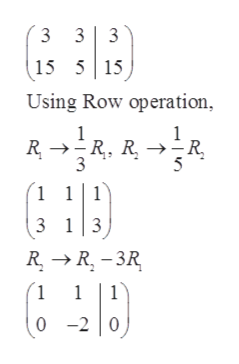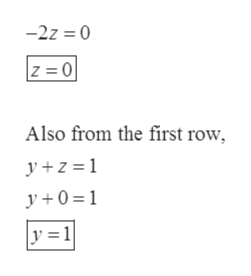# Use Gaussian elimination to solve the given system of equations, 3y+3z=3 and 15y+5z=15.Select the correct answer below: (0,1) (5,0) (1,0) infinitely many solutions no solutions

Question
6 views
Use Gaussian elimination to solve the given system of equations, 3y+3z=3 and 15y+5z=15.

(0,1)

(5,0)

(1,0)

infinitely many solutions

no solutions
check_circle

Step 1

Given:

Step 2

Now making the augment matrix for the given set of equations,help_outlineImage Transcriptionclose3 3 3 15 515 Using Row operation R R, R R 1 11 3 13 RR3R 1 1 1 -2 |0) fullscreen
Step 3

Now using the above echelon form ma...help_outlineImage Transcriptionclose-2z 0 Z 0 Also from the first row y z=1 y0=1 y 1 fullscreen

### Want to see the full answer?

See Solution

#### Want to see this answer and more?

Solutions are written by subject experts who are available 24/7. Questions are typically answered within 1 hour.*

See Solution
*Response times may vary by subject and question.
Tagged in